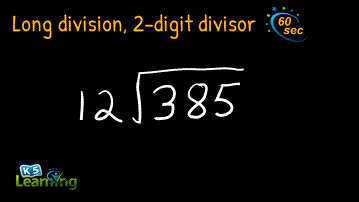# Videos Solve Division Problems in Sixty Seconds

Our new division videos demonstrate basic division skills. The individual videos demonstrate a division concept using a division example. We keep each video concise; each of them solves a question in less than 60 seconds.

## Simple division video

The emphasis in this video is on equal groups, as we show you how to divide 6 carrots amongst 3 friends.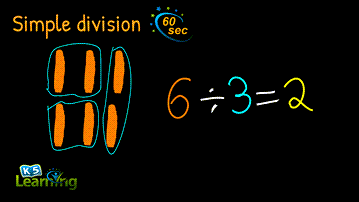## Simple division with remainder

This video shows 7 carrots divided amongst 3 friends, leaving 1 remainder.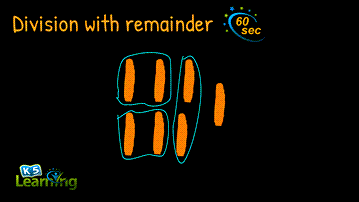## Video of dividing numbers that end in zeros

This video shows students how to divide numbers with a zero or zeros on the end. The example used is 60/3 and 600/3.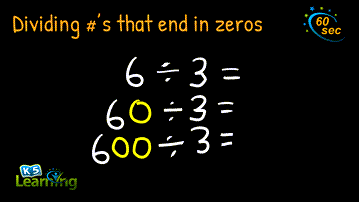## Introduction to long division example

This video introduces students to long division with an example of numbers under 100 and no remainder.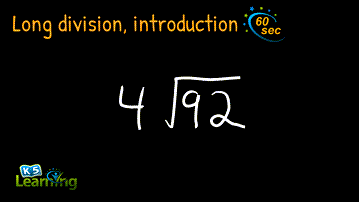## Long division with remainder video

This video takes the long division example one step further, using an example with remainder.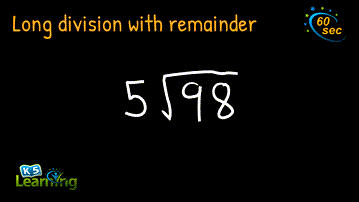## Long division with no remainder example

Now, we tackle a larger number for this long division exercise with no remainder.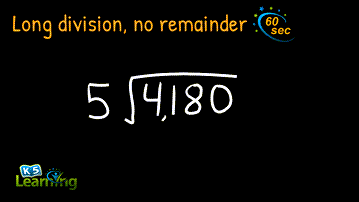## Long division with remainder video

This video shows a harder long division exercise with remainder.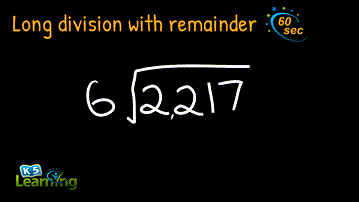## Video of long division with a 2-digit divisor

Here’s an even harder example, where the divisor is a two-digit number.